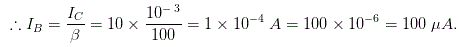# MCQs on Analog Electronics

##### Page 29 of 33. Go to page 1 2 3 4 5 6 7 8 9 10 11 12 13 14 15 16 17 18 19 20 21 22 23 24 25 26 27 28 29 30 31 32 33
01․ The fact that there are many free electrons in a transistor emitter region means the emitter is
lightly doped.
heavily doped.
undoped.
none of the above.

By increasing the emitter junction efficiently by providing heavy doped substrates, the electrons can be transferred majority of electrons which is emitted from emitter to collector.

02․ In a normally biased npn transistor, the electrons in the emitter have enough energy to overcome the barrier potential of the
base - emitter junction.
base - collector junction.
collector - base junction.
recombination path.

The flow of the electrons from the collector to emitter is controlled by the base to emitter electrons which has a certain potential energy. We know that the electron from the collector to emitter is very strong. There is a chance of affecting the base emitter junction by the heavy potential energy from the collector electron. So, the electrons in the emitter should have enough energy to overcome the barrier potential of the base emitter junction.

03․ What is the most important fact about the collector current?
It is measured in milliampere.
It equals the base current divided by the current gain.
It is small.
It approximately equals the emitter current.

According to the KCL, the emitter current is the summation of the base current and the collector current. Since, the base current is very less than the collector current. So, it is neglected. Hence, the emitter current is approximately equal to the collector current.

04․ In a pnp transistor, the major carriers in the emitter are
free electrons.
holes.
neither.
both.

In an NPN transistor, the majority current carriers are electrons. In a PNP transistor, the majority current carriers are holes.

05․ If the current gain is 100 and the collector current is 10 mA, the base current is
10 µA.
100 µA.
1 A.
10 A.

We know that the collector current IC = βIB.06․ The base - emitter voltage is usually
less than the base supply voltage.
equal to the base supply voltage.
more than the base supply voltage.
none of these.

The required base emitter voltage for a transistor is 0.7 Volts. Since, it is typically less than the input voltage. Here, the input voltage is base supply voltage. So, base emitter voltage is always less than the base voltage.

07․ The collector - emitter voltage is usually
less than the collector supply voltage.
equal to the collector supply voltage.
more than the collector supply voltage.
none of these.

There is a voltage drop across the collector resistance. It draws the voltage drop from the input voltage or collector supply voltage. So, the collector emitter voltage is subtraction of the collector supply voltage and the voltage across the collector resistance. Thus, the collector emitter voltage is always less than the collector supply voltage.

08․ The power dissipated by a transistor approximately equals the collector current times
base - emitter voltage.
collector - emitter voltage.
base supply voltage.
0.7 V.

The power dissipated by a transistor is calculated by multiplying the collector current and the collector emitter voltage. The power dissipation is nothing but the output power than can be consumed by the transistor. Thus, the output power (power dissipation) is calculated by the above method.

09․ A small collector current with zero base current is caused by the leakage current of the
emitter diode.
collector diode.
base diode.
transistor.

This thing happens in the cut-off condition. During this time, the base current is zero. In this period, the emitter depletion region is very much narrower than the collector depletion region because of forward bias at the collector and higher doping levels. Hence, there would be a small leakage current flow via collector diode. By this leakage current, the small collector current is generated.

10․ If the base current is 100 mA and the current gain is 30, the emitter current is
3.33 mA.
3 A.
3.1 A.
10 A.

The emitter current (IE) = base current (IB) + collector current (IC). Here, collector current = base current (IB) X current gain (β) = 100 X 10-3 X 30 = 3 Amps. Now, emitter current (IE) = 3 + (100 X 10-3) = 3.1 Amps.

<<<2728293031>>>